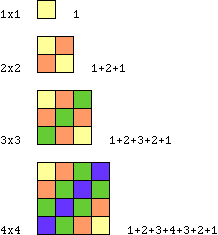Date: Mon, 14 Sep 1998 18:57:21 -0600 (CST)
Subject: Math

Name: Krystin

Level: Secondary

Question:
1=1x1
1+2+1=2x2
1+2+3+2+1=3x3

draw a series of diagrams to illustrate the above pattern

Hi KrystinCheers,
Penny

Go to Math Central